Please wait a minute...
 Chin. Phys. B, 2020, Vol. 29(11): 114101    DOI: 10.1088/1674-1056/abab71
 ELECTROMAGNETISM, OPTICS, ACOUSTICS, HEAT TRANSFER, CLASSICAL MECHANICS, AND FLUID DYNAMICS Prev   Next

# Effect of patterned hydrodynamic slip on electromagnetohydrodynamic flow in parallel plate microchannel

Chun-Hong Yang(杨春红) and Yong-Jun Jian(菅永军)
School of Mathematical Science, Inner Mongolia University, Hohhot 010021, China
 Abstract  A fully developed electromagnetohydrodynamic (EMHD) flow through a microchannel with patterned hydrodynamic slippage on the channel wall is studied. The flow is driven by the Lorentz force which originates from the interaction between an externally imposed lateral electric field and a perpendicular magnetic field. The governing equations for the velocity with patterned slip boundary conditions are solved analytically by perturbation techniques under the assumption of small Reynolds number Re. In addition, the numerical solutions for the velocity are obtained by using the finite-difference method, and they are found to be in good agreement with the analytical solutions within admissible parameter range. The effects of different parameters on the velocity and volume flow rate due to patterned hydrodynamic slippage are discussed in detail, including wave-number K, Hartmann number Ha, amplitude δ of the patterned slip length, and normalized electric field strength S. The results show that patterned slippage over microchannel walls can induce transverse flows, which will increase the mixing rates in microfluidic devices. In addition, we also find that precise flow control can be achieved by controlling the magnetic flux and the wave-number and also by well choosing the electric field intensity. Our analysis can be used for designing the efficient micro-fluidic mixers. Keywords:  electromagnetohydrodynamic (EMHD) flow      patterned slip wall Received:  03 April 2020      Revised:  24 May 2020      Accepted manuscript online:  01 August 2020 Fund: the National Natural Science Foundation of China (Grant Nos. 11772162 and 11472140), the Natural Science Foundation of Inner Mongolia Autonomous Region, China (Grant Nos. 2016MS0106 and 2018MS01015), and the Inner Mongolia Grassland Talent, China (Grant No. 12000-12102013). Corresponding Authors:  †Corresponding author. E-mail: jianyj@imu.edu.cn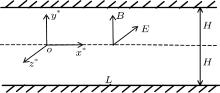Fig. 1.  Schematic diagram of physical modeling.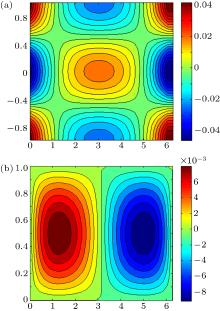Fig. 2.  Contours plots for dimensionless velocity variations of (a) axial velocity u1, (b) vertical velocity v1 with a cosine wave variation in slip length on wall at Re = 0.01, Ha = 1, S = 1, Ω = 0, δ = 0.1, and K = 1.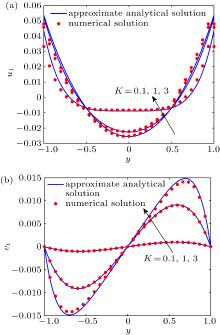Fig. 3.  Comparison between analytical and numerical solutions for the first-order (a) axial velocity u1 and (b) vertical velocity v1 at three different values of wave-number K (x = 0.0628, Re = 0.01, Ha = 1, S = 1, Ω = 0, δ = 0.1).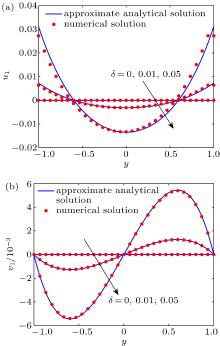Fig. 4.  First-order velocity distributions for different values of non-dimensional slip length δ at K = 1 (x = 0.0628, Re = 0.01, Ha = 1, S = 1, Ω = 0), sowing (a) axial velocity u1 and (b) vertical velocity v1 varying with y.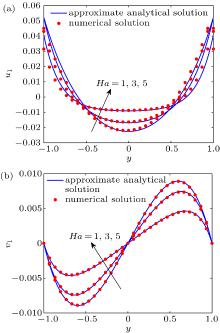Fig. 5.  First-order velocity distributions for different values of Hartmann number Ha (x = 0.2096, Re = 0.01, S = 1, Ω = 0, K = 1, δ = 0.1, α = 0.01), showing (a) axial velocity u1 and (b) vertical velocity v1 versus y.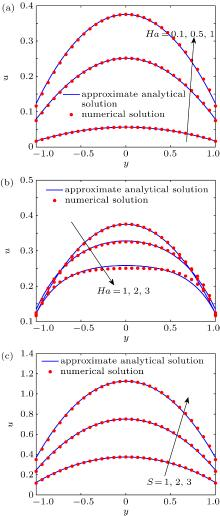Fig. 6.  Variations of total axial velocity u = u0 + α u1 with y for different values of Hartmann number Ha and electric field strength S: [(a), (b)] S = 1 and (c) Ha = 0.1 at x = 0.2096, Re = 0.01, Ω = 0, K = 1, δ = 0.1, α = 0.01.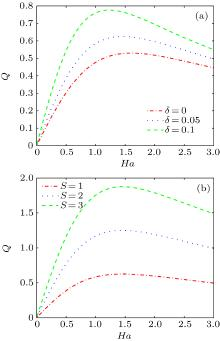Fig. 7.  Variations of total axial volume flow rate with Ha for different values of slip length δ and electric field strength S: (a) S = 1 and (b) δ = 0.1 at x = 0.2096, Re = 0.01, Ω = 0, K = 1, and α = 0.01.